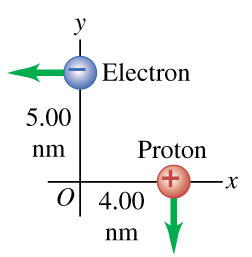# Problem: An electron and a proton are each moving at 795 km/s in perpendicular paths as shown in (Figure 1) .Part AFind the magnitude of the total electrical force that the electron exerts on the proton.Part BFind the direction of the total electrical force that the electron exerts on the proton counter clockwise from the positive x axis.

###### FREE Expert Solution

Part A

Coulomb's law:

r = 6.403 × 10-9 m

82% (472 ratings)###### Problem Details

An electron and a proton are each moving at 795 km/s in perpendicular paths as shown in (Figure 1) .Part A

Find the magnitude of the total electrical force that the electron exerts on the proton.

Part B

Find the direction of the total electrical force that the electron exerts on the proton counter clockwise from the positive x axis.